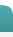|  Login Web SiteOhm's Law & DC PowerOhm's Law E = I * R I = E / R R = E / I where E = voltage in volts, I = current in amps, and R = resistance in ohms    DC Power P = E * I P = (E ** 2) / R P = (I ** 2) * R where P = power in wattsTerms Of Use  |  Privacy StatementCopyright © 2009-2012 by Automation Technologies, Inc.
health watch